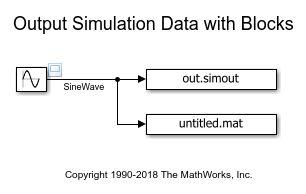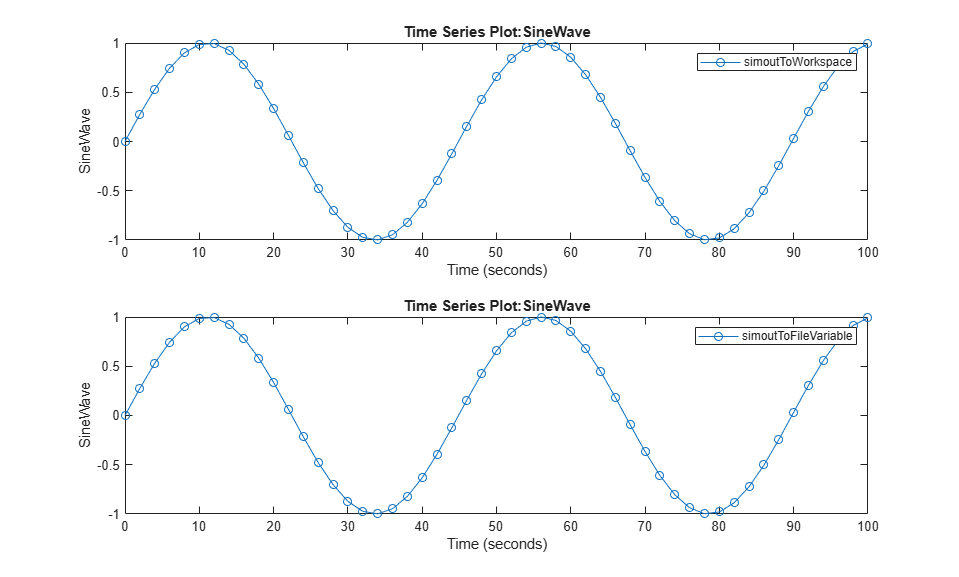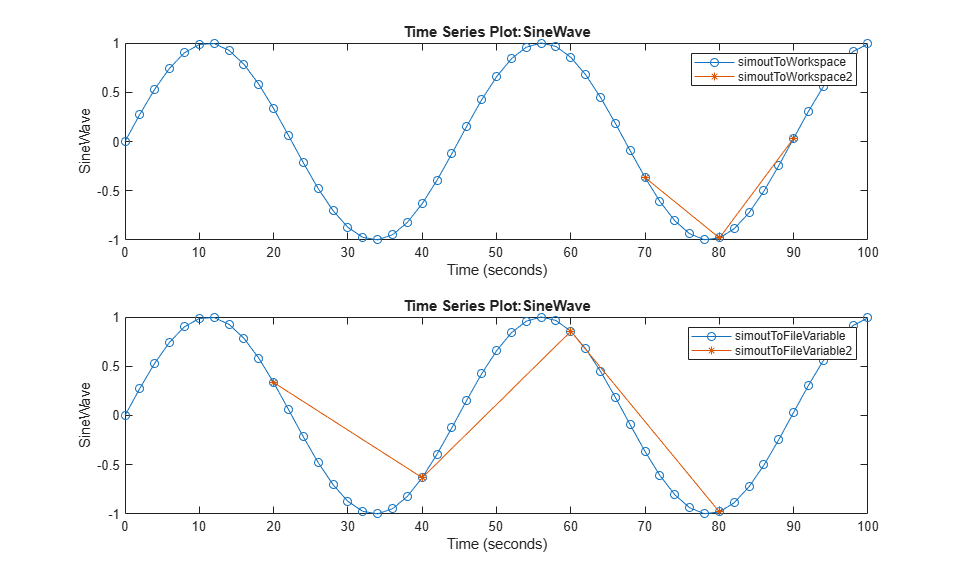Documentation

This is machine translation

Mouseover text to see original. Click the button below to return to the English version of the page.

Output Simulation Data with Blocks

This example shows how To Workspace and To File blocks write data to the workspace and to a file respectively.

Open Example Model

```open_system('ex_ToWorkspace_ToFile'); ```Simulate with Default Parameter Values

1. To name the output variables and file, modify the Variable name and File name block parameter values by using the Block Parameters dialog boxes or the command line.

```set_param('ex_ToWorkspace_ToFile/To Workspace',... 'VariableName','simoutToWorkspace') set_param('ex_ToWorkspace_ToFile/To File',... 'FileName','simoutToFile.mat',... 'MatrixName','simoutToFileVariable') ```

2. Simulate the model.

```out = sim('ex_ToWorkspace_ToFile'); ```

3. To view the input signal for the To Workspace and To File blocks, open the scope viewer.

4. To access the data stored by the To File block, load the output file.

```load('simoutToFile.mat') ```

5. Plot the data stored by the To Workspace and To File blocks.

```subplot(2,1,1) plot(out.simoutToWorkspace,'-o') legend('simoutToWorkspace') subplot(2,1,2) plot(simoutToFileVariable,'-o') legend('simoutToFileVariable') ```As shown by the plots, the data stored by each block is the same given the default block parameter values.

Simulate with Custom Parameter Values

1. To keep the data from the previous simulation, specify new names for the output variables and file.

```set_param('ex_ToWorkspace_ToFile/To Workspace',... 'VariableName','simoutToWorkspace2') set_param('ex_ToWorkspace_ToFile/To File',... 'FileName','simoutToFile2.mat',... 'MatrixName','simoutToFileVariable2') ```

2. To change the amount of data collected, modify the Limit data points to last, Decimation, and Sample time block parameter values.

```set_param('ex_ToWorkspace_ToFile/To Workspace',... 'MaxDataPoints','3',... 'Decimation','20',... 'SampleTime','0.5') set_param('ex_ToWorkspace_ToFile/To File',... 'Decimation','20',... 'SampleTime','1') ```

The To File block does not provide the option to limit data points to the last data points collected.

3. Select Single simulation output, then modify the Logging intervals configuration parameter value.

```set_param('ex_ToWorkspace_ToFile',... 'ReturnWorkspaceOutputs','on',... 'LoggingIntervals','[20,90]') ```

4. Simulate the model.

```out = sim('ex_ToWorkspace_ToFile'); ```

5. To access the data stored by the To File block, load the output file.

```load('simoutToFile2.mat') ```

6. Plot the data stored by the To Workspace and To File blocks.

```subplot(2,1,1) hold on plot(out.simoutToWorkspace2,'-*','DisplayName','simoutToWorkspace2') hold off subplot(2,1,2) hold on plot(simoutToFileVariable2,'-*','DisplayName','simoutToFileVariable2') hold off ```In this example, the To Workspace block collects data at 20, 30, 40, ..., 90 seconds. The data represents every 20th sample time within the logging intervals. When the simulation is completed or paused, the To Workspace block writes only the last three collected sample points to the workspace: 70, 80, and 90 seconds.

The To File block collects data at 20, 40, 60, and 80 seconds. The data similarly represents every 20th sample time within the logging intervals. However, the sample time for the To File block is double the sample time for the To Workspace block.